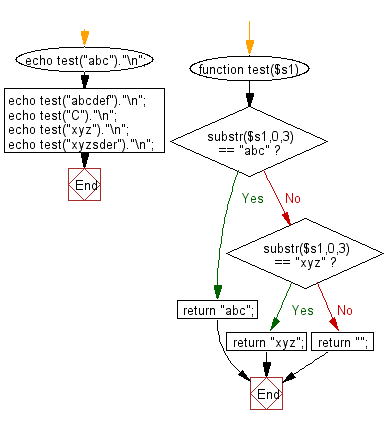﻿ PHP Exercises: Check if a given string begins with 'abc' or 'xyz' - w3resource# PHP Exercises: Check if a given string begins with 'abc' or 'xyz'

## PHP Basic Algorithm: Exercise-79 with Solution

Write a PHP program to check if a given string begins with 'abc' or 'xyz'. If the string begins with 'abc' or 'xyz' return 'abc' or 'xyz' otherwise return the empty string.

Sample Solution:

PHP Code :

``````<?php
function test(\$s1)
{
if (substr(\$s1,0,3) == "abc")
{
return "abc";
}
else if (substr(\$s1,0,3) == "xyz")
{
return "xyz";
}
else
{
return "";
}
}

echo test("abc")."\n";
echo test("abcdef")."\n";
echo test("C")."\n";
echo test("xyz")."\n";
echo test("xyzsder")."\n";
``````

Sample Output:

```abc
abc

xyz
xyz
```

Flowchart:PHP Code Editor:

What is the difficulty level of this exercise?

﻿

## PHP: Tips of the Day

\$_REQUEST: This SuperGlobal Variable is used to collect data submitted by a HTML Form

Example:

This code save in a php file and run in the browser.

```<!DOCTYPE html>
<html>
<body>

<form method="post" action="<?php echo \$_SERVER['PHP_SELF'];?>">
NAME: <input type="text" name="user">
<button type="submit">SUBMIT</button>
</form>
<?php
if (\$_SERVER["REQUEST_METHOD"] == "POST") {
\$name = htmlspecialchars(\$_REQUEST['user']);
if(empty(\$name)){
echo "Name is empty";
} else {
echo \$name;
}
}
?>
</body>
</html>
```

Output:

```Owen
```